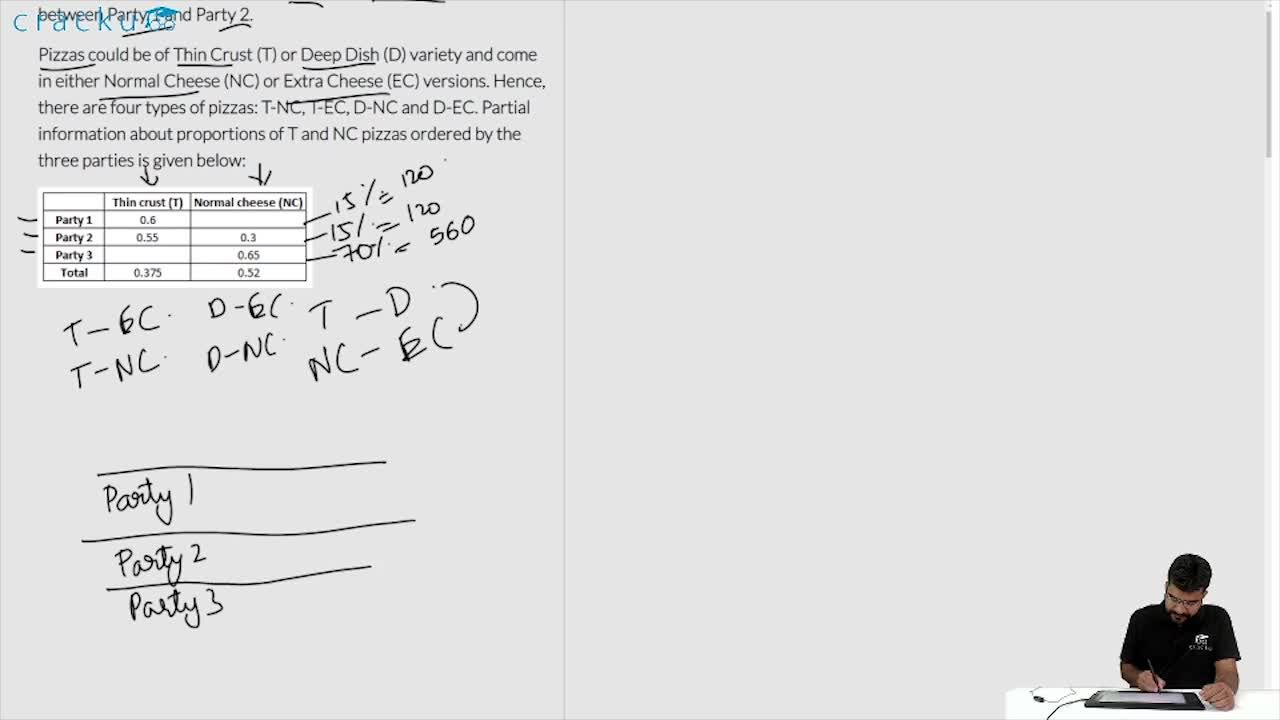### CAT 2017 Question Paper (Shift-2) Question 36

Instructions

Funky Pizzeria was required to supply pizzas to three different parties. The total number of pizzas it had to deliver was 800, 70% of which were to be delivered to Party 3 and the rest equally divided between Party 1 and Party 2.

Pizzas could be of Thin Crust (T) or Deep Dish (D) variety and come in either Normal Cheese (NC) or Extra Cheese (EC) versions. Hence, there are four types of pizzas: T-NC, T-EC, D-NC and D-EC. Partial information about proportions of T and NC pizzas ordered by the three parties is given below:

Question 36

# How many Normal Cheese pizzas were required to be delivered to Party 1?

Solution

We are given that Party 3 received 70% of total pizzas,therefore, number of pizzas received by Party 3 = $$\frac{70}{100}\times 800$$ = 560

Remaining 240 pizzas are equally divided among party 1 and party 2 hence we can say that each of Party 1 and Party 2 received 120 pizzas.

We know that all of the pizza can be classified into a total of 4 types. Hence, on drawing a table which can accommodate all of the cases:

Total number of Thin Crust pizzas = 0.375*800 = 300. Therefore, total number of Deep Dish pizzas = 800 - 300 = 500.

Out of 120 pizzas that Party 1 received, 60% were of Thin Crust type hence, total number of Thin Crust pizza received by Party 1 = 0.6*120 = 72. Consequently Party 1, must have received 42 Deep Dish type pizzas.

Out of 120 pizzas that Party 2 received, 55% were of Thin Crust type hence, total number of Thin Crust pizza received by Party 2 = 0.55*120 = 66. Consequently Party 1, must have received 54 Deep Dish type pizzas.

Therefore, total number of Thin Crust pizzas ordered by Party 3 = Total Thin Crust pizzas ordered - Thin Crust pizzas ordered by Party 1 - Thin Crust pizzas ordered by Party 2

$$\Rightarrow$$ 300 - 72 - 66 = 162

Hence number of Deep Dish type of pizzas order by Party 3 = 560 - 162 = 398

Total number of Normal Cheese pizzas require to be delivered = 0.52*800 = 416
Number of  Normal Cheese pizzas require to be delivered to Party 2 = 0.3*120 = 36
Number of  Normal Cheese pizzas require to be delivered to Party 3 = 0.65*560 = 364

Therefore, total number of Normal Cheese pizzas  require to be delivered to Party 1 = Total Normal Cheese pizzas to be delivered - Normal Cheese pizzas require to be delivered to Party 2 - Normal Cheese pizzas require to be delivered to Party 3

$$\Rightarrow$$ 416 - 36 - 364 = 16

Hence, option C is the correct answer.

### View Video Solution